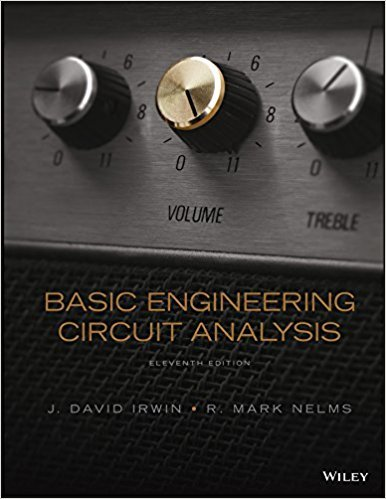×
Get Full Access to Basic Engineering Circuit Analysis - 11 Edition - Chapter 2 - Problem 2.4
Get Full Access to Basic Engineering Circuit Analysis - 11 Edition - Chapter 2 - Problem 2.4

×

# In the network in Fig. P2.4, the power absorbed by Gx isISBN: 9781118539293 159

## Solution for problem 2.4 Chapter 2

Basic Engineering Circuit Analysis | 11th Edition

• Textbook Solutions
• 2901 Step-by-step solutions solved by professors and subject experts
• Get 24/7 help from StudySoup virtual teaching assistantsBasic Engineering Circuit Analysis | 11th Edition

4 5 1 338 Reviews
23
0
Problem 2.4

In the network in Fig. P2.4, the power absorbed by Gx is 20 mW. Find Gx. Figure P2.4

Step-by-Step Solution:
Step 1 of 3

Module 3 D : Clocked Synchronous State Machine We are going to talk about two different state machine structures known as Moore and Mealy Machines. State machine is a generic name given to sequential circuits. CLOCKED indicates that the flip flops employ a clock input. Synchronous meaning that all the flip flops change state together. Analysis means to analyze the behavior using steps : constructing the PS – NS equations, drawing the state transition diagram and the timing chart. State Machine structure consists of three blocks : 1) Next state logic – combinational circuity that provides the excitation necessary to transition to the next state based on the current inputs 2) output logic -- combinational circuitry that uses the current state to determine th

Step 2 of 3

Step 3 of 3

##### ISBN: 9781118539293

Unlock Textbook Solution